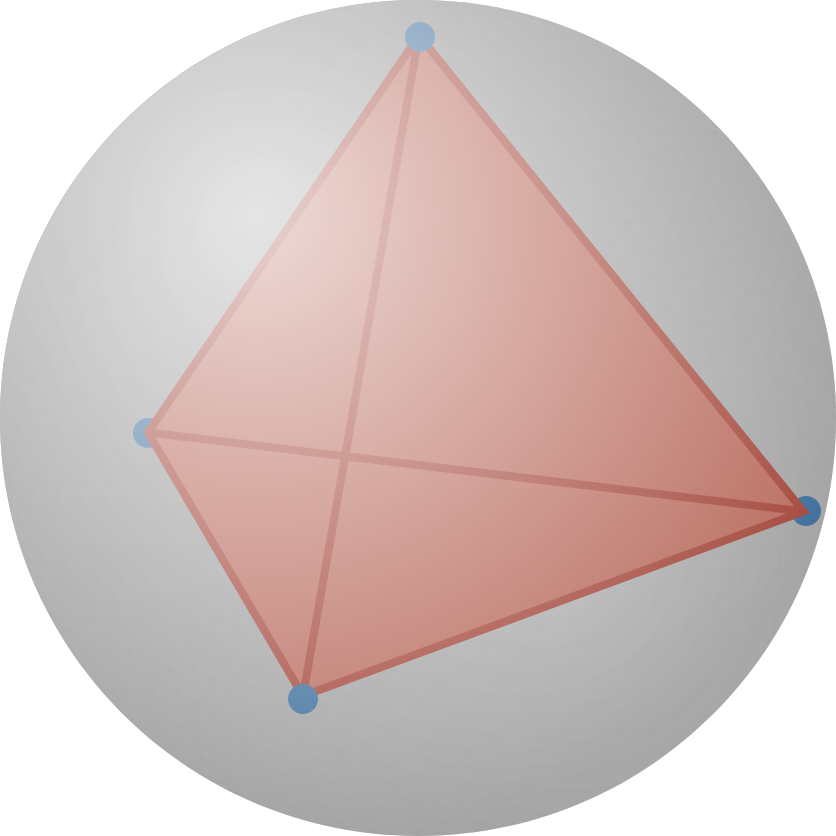# Christmas Streak 15/88: This Is A Bit Repulsive

Geometry Level 3Tom has a set of particles that repel each other with a force of $F(r)$ whose strength is proportional to $\frac{1}{r^2},$ where $r$ is the distance of separation between any pair of particles.

If Tom puts 8 of these particles into a rigid, frictionless sphere and allows them to come to rest, they'll align to the vertices of a certain $n$-faced polyhedron.

What is $n?$

For example, if Tom puts 4 of the particles into the sphere, they'll come to rest at the vertices of a regular tetrahedron, as shown.

×